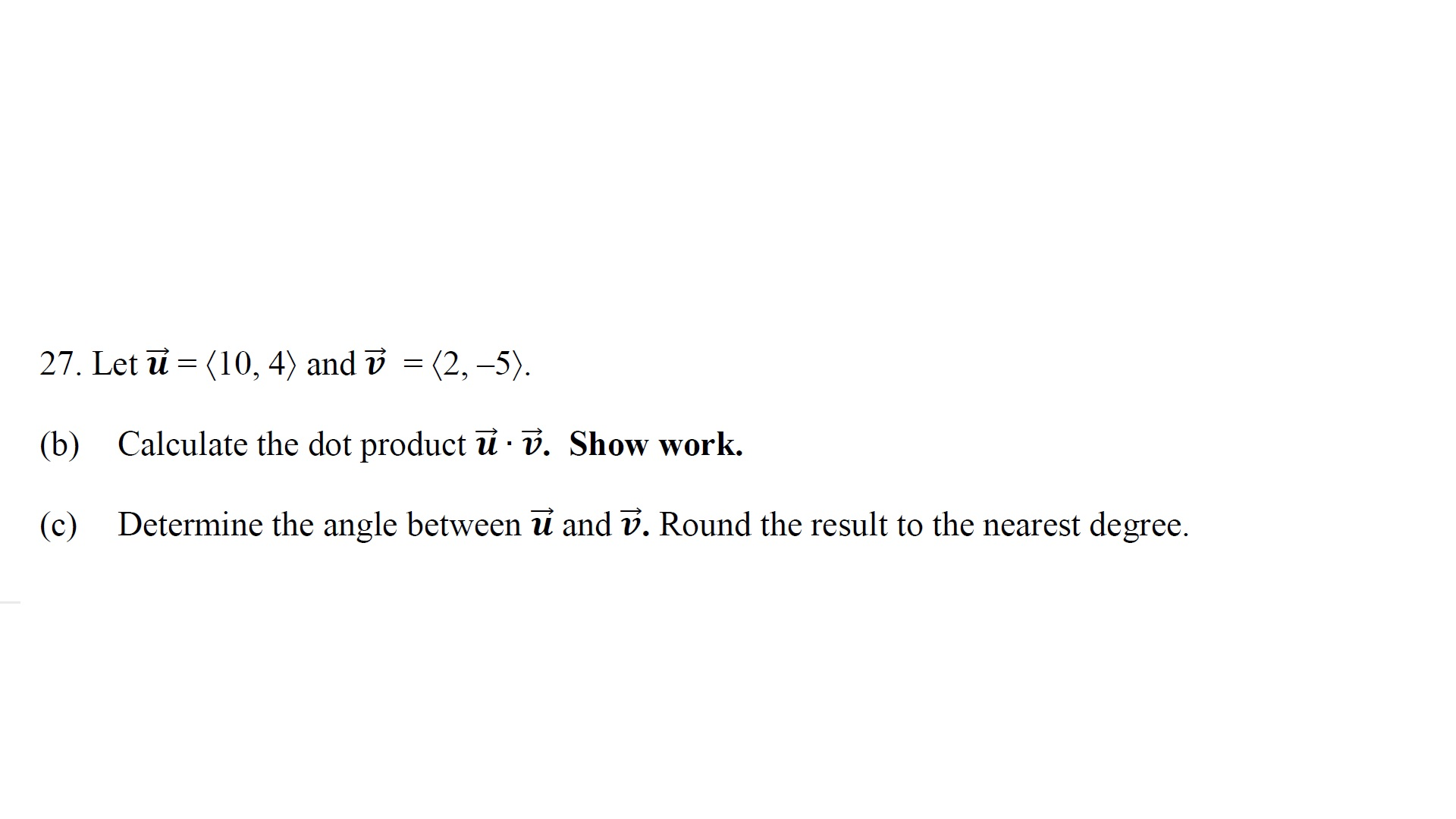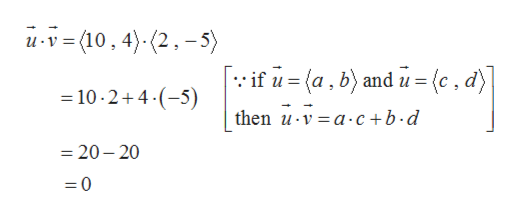# 27. Let u (10, 4) and v = (2, -5)Calculate the dot product u v. Show work.(b)Determine the angle between u and v. Round the result to the nearest degree(c)

Question
2 viewshelp_outlineImage Transcriptionclose27. Let u (10, 4) and v = (2, -5) Calculate the dot product u v. Show work. (b) Determine the angle between u and v. Round the result to the nearest degree (c) fullscreen
check_circle

Step 1

Given,

Step 2

b). The dot pro...help_outlineImage Transcriptioncloseu-v=(10,4)(2,-5) [if u= (a, b) and u = {c , d) 10.2+4.(5) then uv a.c+b-d =20-20 =0 fullscreen

### Want to see the full answer?

See Solution

#### Want to see this answer and more?

Solutions are written by subject experts who are available 24/7. Questions are typically answered within 1 hour.*

See Solution
*Response times may vary by subject and question.
Tagged in

### Other